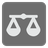Can Balance
Simple
English

Each item in the list of items is considered to be a physical weight, and guaranteed to be a positive integer. Your task is to find and return a fulcrum position in this list so that when balanced on that position, the total torque of the items to the left of that position equals the total torque of the items to the right of that position. (The item on the fulcrum is assumed to be centered symmetrically on both sides, and therefore doesn’t participate in the torque calculation.)

As taught in any introductory physics textbook, the torque of an item with respect to the fulcrum equals its weight times its distance from the fulcrum. If a fulcrum position exists, return that position, otherwise return -1 to indicate that the given items cannot be balanced, at least not without being rearranged.

Input: The iterable of positive integers.

Output: Int.

Example:

```# 6*2 + 1*1 == 5*1 + 4*2
can_balance([6, 1, 10, 5, 4]) == 2
# 10*1 == 3*1 + 2*2 + 1*3
can_balance([10, 3, 3, 2, 1]) == 1
```

The mission was taken from Python CCPS 109 Fall 2018. It’s being taught for Ryerson Chang School of Continuing Education by Ilkka Kokkarinen